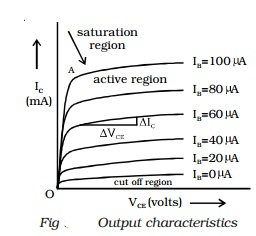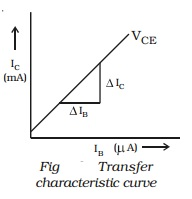Home | | Physics | Characteristics of an NPN transistor in common emitter configuration

# Characteristics of an NPN transistor in common emitter configurationThe three important characteristics of a transistor in any mode are (i) input characteristics (ii) output characteristics and (iii) transfer characterstics. The circuit to study the characteristic curves of NPN transistor in common emitter mode is as shown in Fig.

Characteristics of an NPN transistor in common emitter configuration

The three important characteristics of a transistor in any mode are (i) input characteristics (ii) output characteristics and (iii) transfer characterstics.

The circuit to study the characteristic curves of NPN transistor in common emitter mode is as shown in Fig.(i) Input characteristics

Input characteristic curve is drawn between the base current (IB) and voltage between base and emitter (VBE), when the voltage between collector and emitter (VCE) is kept constant at a particular value. VBE is increased in suitable equal steps and corresponding base current is noted. The procedure is repeated for different values of VCE.

IB values are plotted against VBE for constant VCE. The input characteristic thus obtained is shown in Fig.The input impedance of the transistor is defined as the ratio of small change in base - emitter voltage to the corresponding change in base current at a given VCE.The input impedance of the transistor is defined as the ratio of small change in base - emitter voltage to the corresponding change in base current at a given VCE.

Input impedance, r1  = (∆VBE/∆IB)VCE

The input impedance of the transistor in CE mode is very high.

(ii) Output characteristics

Output characteristic curves are drawn between IC and VCE, when IB is kept constant at a particular value.

The base current IB is kept at a constant value, by adjusting the base emitter voltage VBE. VCE is increased in suitable equal steps and the corresponding collector current is noted. The procedure is repeated for different values of IB. Now, Ic versus VCE curves are drawn for different values of IB. The output characteristics thus obtained are represented in Fig. The three regions of the characteristics can be discussed as follows :

Saturation region : The initial part of the curve (ohmic region, OA) is called saturation region. (i.e) The region in between the origin and knee point. (Knee point is the point, where Ic is about to become a constant).

Cut off region : There is very small collector current in the transistor, even when the base current is zero (IB = 0). In the output characteristics, the region below the curve for IB = 0 is called cut off region. Below the cut off region, the transistor does not function.

Active region : The central region of the curves is called active region. In the active region, the curves are uniform. In this region, E-B junction is forward biased and C-B junction is reverse biased.The output impedance ro is defined as the ratio of variation in the collector emitter voltage to the corresponding variation in the collector current at a constant base current in the active region of the transistor characteristic curves.

output impedence, ro = (∆VCE/ ∆IC)IB

The output impedance of a transistor in CE mode is low.

(iii) Transfer characteristics

The transfer characteric curve is drawn between IC and IB, when VCE is kept constant at a particular value. The base current IB is increased in suitable steps and the collector current IC is noted down for each value of IB. The transfer characteristic curve is shown in Fig.

The current gain is defined as the ratio of a small change in the collector current to the corresponding change in the base current at a constant VCE.

Current gain, β = (∆IC/ ∆IB)VcE

The common emitter configuration has high input impedance, low output impedance and higher current gain when compared with common base configuration.Study Material, Lecturing Notes, Assignment, Reference, Wiki description explanation, brief detail
11th 12th std standard Class Physics sciense Higher secondary school College Notes : Characteristics of an NPN transistor in common emitter configuration |